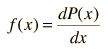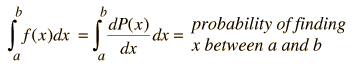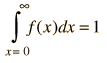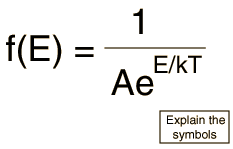# Basic Probability

The probability for a given event can be thought of as the ratio of the number of ways that event can happen divided by the number of ways that any possible outcome could happen. The concept of probability is one of the foundations of general statistics. The likelihood of a particular outcome among the set of possible outcomes is expressed by a number from 0 to 1, with 0 representing an impossible outcome and 1 representing the absolute certainty of that outcome. If we identify the set of all possible outcomes as the "sample space" and denote it by S, and label the desired event as E, then the probability for event E can be writtenIn the probability of a throw of a pair of dice, bet on the number 7 since it is the most probable. There are six ways to throw a 7, out of 36 possible outcomes for a throw. The probability is thenThe idea of an "event" is a very general one. Suppose you draw five cards from a standard deck of 52 playing cards, and you want to calculate the probability that all five cards are hearts. This desired event brings in the idea of a combination. The number of ways you can pick five hearts, without regard to which hearts or which order, is given by the combinationwhile the total number of possible outcomes is given by the much larger combinationThe same basic probability expression is used, but it takes the formSo drawing a five-card hand of a single selected suit is a rare event with a probability of about one in 2000.

If you want the probabability that any one of a number of disjoint events will occur, the probabilities of the single events can be added. For example, the probability of drawing five cards of any one suit is the sum of four equal probabilities, and four times as likely. In boolean language, if the events are related by a logical OR, then the probabilities add. The probability of 5 hearts OR 5 clubs OR 5 diamonds OR 5 spades is approximately .002 or 1 out of 500, four times the probability of drawing 5 hearts.

If the events are related by a logical AND, the resultant probability is the product of the individual probabilities. If you want the probability of throwing a 7 with a pair of dice AND throwing another 7 on the second throw, then the probability would be the productA common misconception about probability is that being "lucky" once will lower the probability that you will be "lucky" again. Probability has no causative effect, so prior events have no influence on the probability for future events. For example:

• Probability of throwing a "2" with a single die: 1/6
• Probability of throwing "2" twice in a row, "2" AND "2": 1/6 x 1/6=1/36
• Probability of throwing a "2" on the next throw: 1/6

The "lucky" circumstance of throwing two "2"s in a row does not make it less likely that you will throw a "2" on the next throw. It is still 1/6.

The expression for probability must be such that the addition of the probabilities for all events must be 1. Constraining the sum of all the probabilities to be 1 is called "normalization". When you calculate the probability by direct counting processes like those discussed above, then the probabilities are always normalized. But when you develop expressions for the probability of events in nature, you must make sure that your probability expression is normalized.

 The probability distribution function
Index

Statistics concepts

 HyperPhysics*****HyperMath R Nave
Go Back

# Probability Distribution Function

Dealing with basic probability as a discrete counting process is satisfactory if you have reasonably small numbers, like throwing dice or picking cards. But if the number of events is very large, as in the distribution of energy among the molecules of a gas, then the probability can be approximated by a continuous variable so that the methods of calculus can be used.

Using the variable x to represent a possible outcome or event, then in the discrete case the basic framework could be summarized as follows:

 For a particular outcome xi P(xi) = probability that xi will be observed ΣiP(xi) =1 (normalization)

If you allow the outcome x to take a continuous range of values, then the probability P(x) takes a different character, since to get a finite result for probability, you must sum the probability over a finite range of x. Since x is a continuous variable, this sum takes the form of an integral. A common practice to define a distribution function as a derivative of the probabilityThe probability of finding outcomes between x=a and x=b can then be expressed asand the normalization condition is thenFor application of probability to physical processes, the use of the distribution function is a very useful strategy. Perhaps the most widely used distribution function in classical physics is the Boltzmann distribution function, which describes the probability of finding particles with an amount of energy E at a given temperature T.Examples of distribution functions
 Physical applications of distribution functions
Index

Statistics concepts

 HyperPhysics*****HyperMath R Nave
Go Back